Published

# Holebot (Pothole Detector)

A fully autonomous pothole detector that updates a visual backend in real-time, and also provides detailed data stored on an SD card.

IntermediateFull instructions provided1,268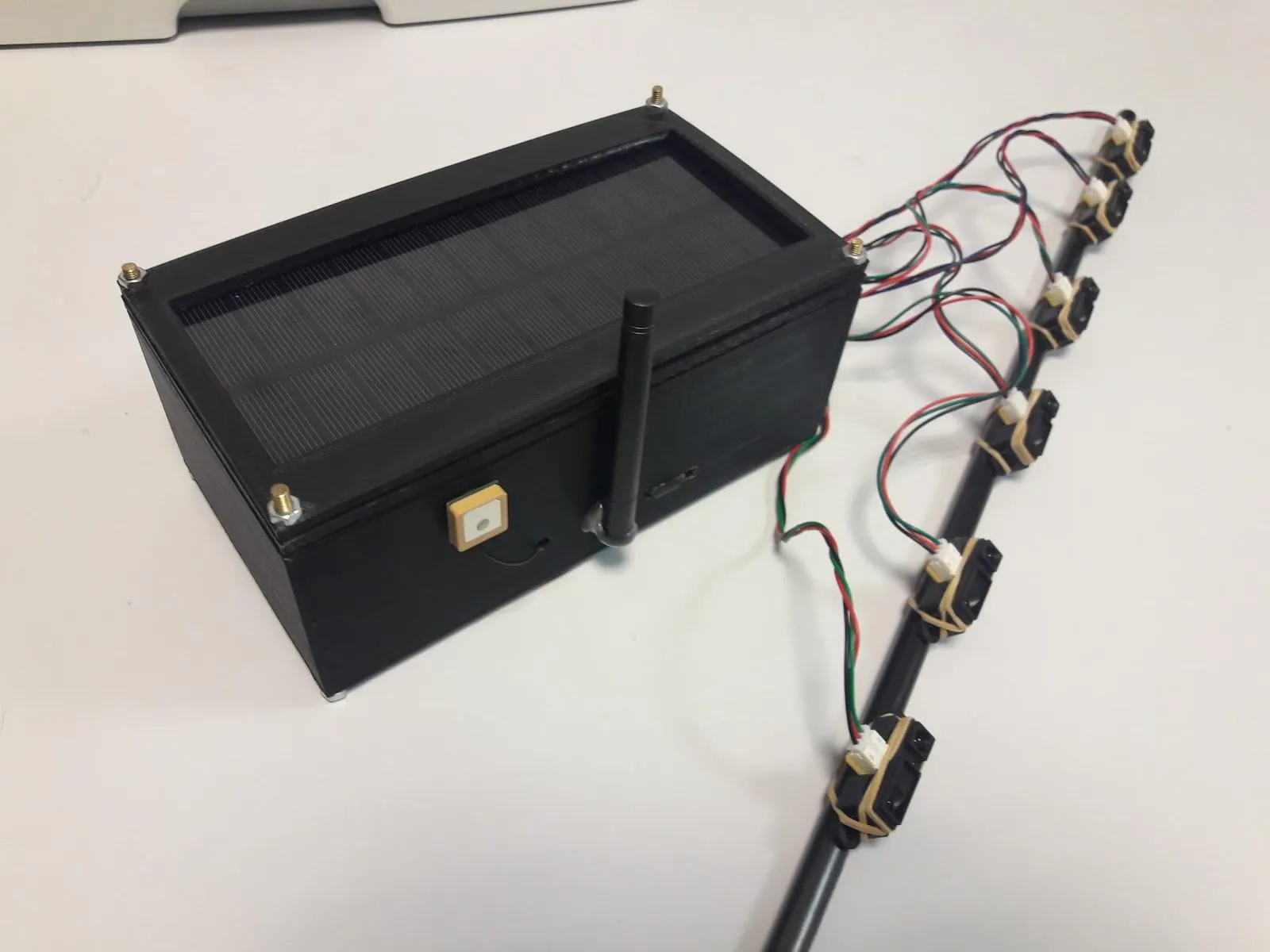## Things used in this project

### Hardware components

 STM32 Nucleo-L432KC
×1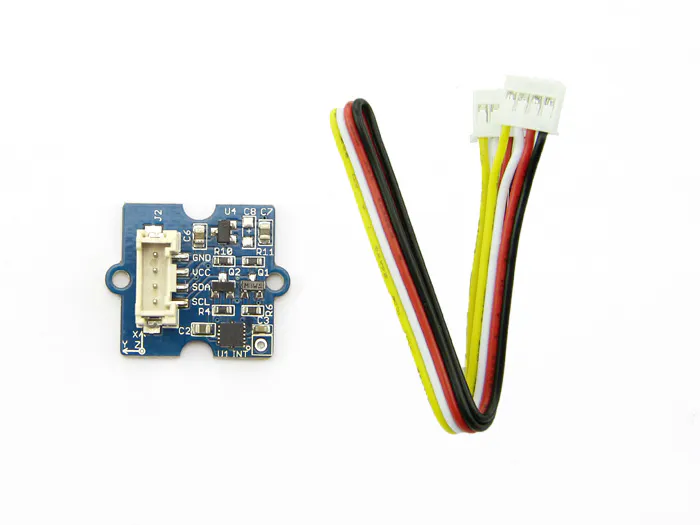Seeed Grove - 3-Axis Digital Accelerometer(±1.5g)
×1
 Seeed Grove GPS
×1
 Sigfox module
×1
 Sharp Infrared Sensor
×6
 DFRobot Micro-SD module
×1
 Li-Ion charger MAX1555DS
×1
 Buck-Boost Converter MCP1252/3
×1
 100 kΩ Resistor
×1
 1 μF Capacitor
×4
 10 μF Capacitor
×2
 Solar cell
×1
 Li-Ion battery
×1
 10 μF Capacitor
×12

### Software apps and online services

 Arm Mbed CompilerMicrosoft Azure
 Microsoft Power BI

## Schematics

### Main PCB schematic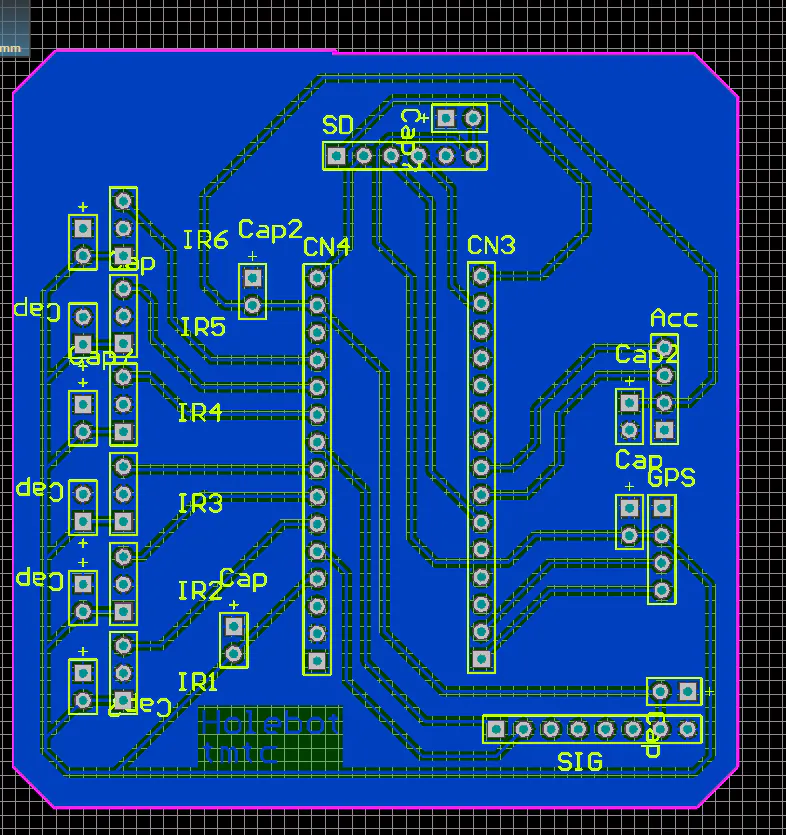### Power supply PCB schematic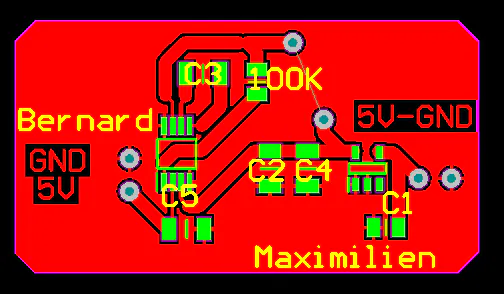### Functional diagram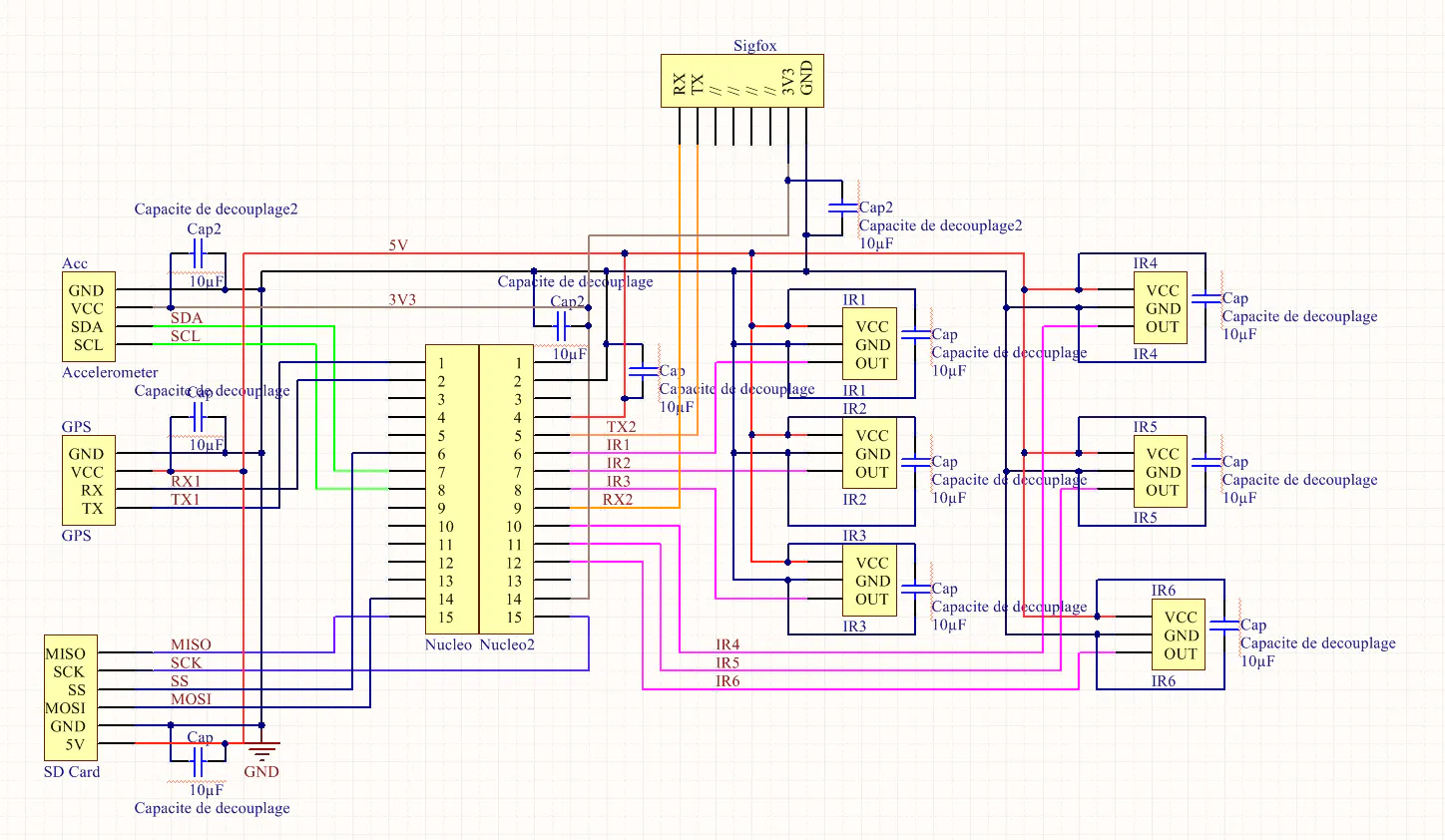### Energy consumption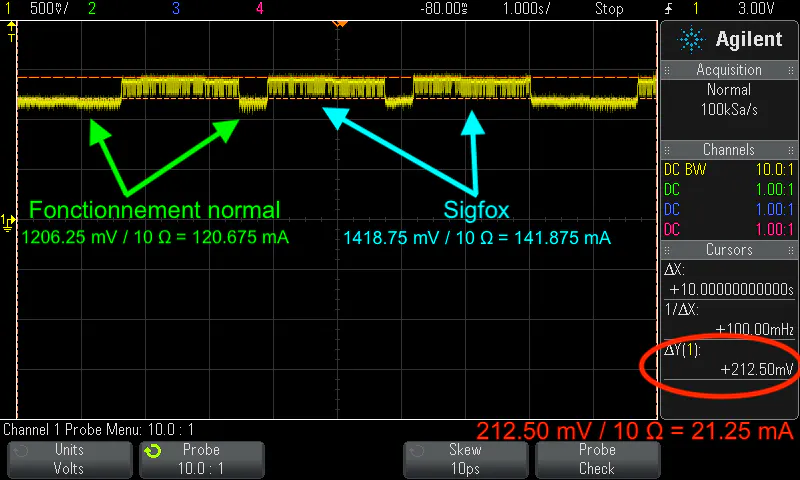### Enclosure 3D Model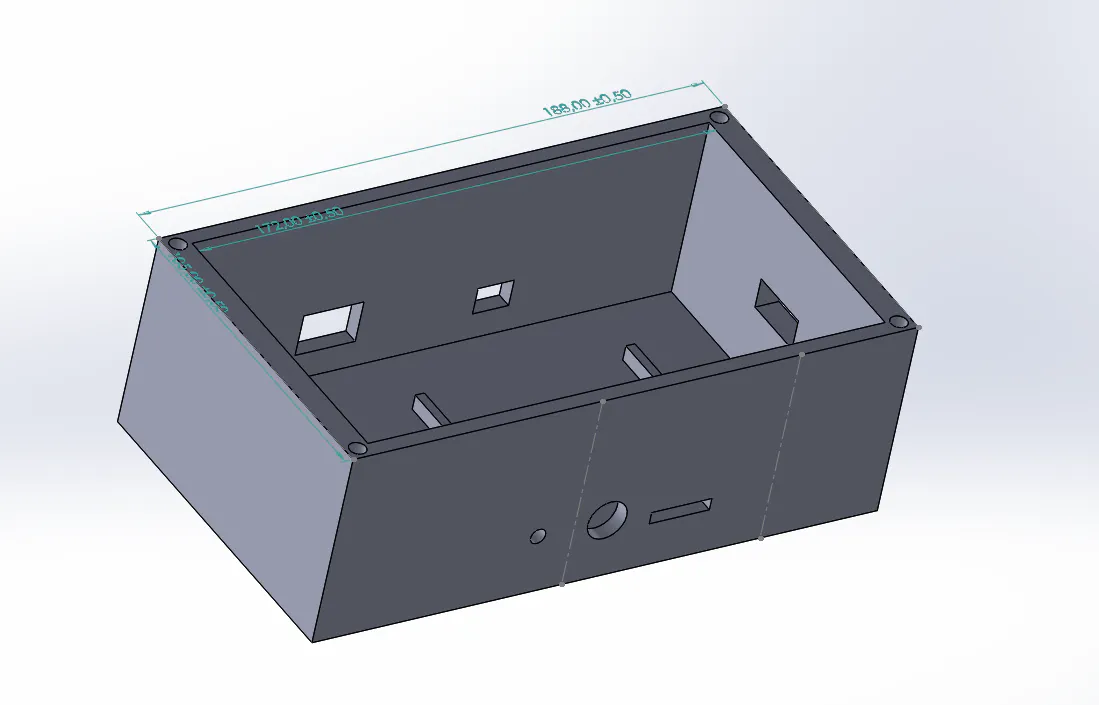## Code

### main.cpp

C/C++
The final Holebot code that runs the entirety of the system
```#include "mbed.h"
#include "GroveGPS.h"
#include <SDBlockDevice.h>
#include <FATFileSystem.h>
#include <string>
#include <cstring>
#include <sstream>
#include <string>

///////////////////////////
//// Various Constants ////
///////////////////////////

#define COOLDOWN 100000                // Cooldown time
#define GPS_SIZE 16                 // Size of GPS position arrays
#define NORMAL_DISTANCE 15          // Normal IR-sensor-to-ground distance (in cm)
#define SENSITIVITY 3               // IR sensor sensitivity (in cm)
Serial gpsSerial(D1, D0, 9600);     // GPS module
Serial sigfox(SERIAL_TX, SERIAL_RX, 9600);  // Telecommunications module
Serial pc(SERIAL_TX, SERIAL_RX, 9600);

// Transistors
DigitalOut line_5V(D7);
DigitalOut line_3V(D8);

SDBlockDevice sd(D11, D12, D13, D3); // SD card reader module
FATFileSystem fs("sd"); // FAT file system to handle SD card

GroveGPS gps;                       // Initialize the Grove GPS
I2C i2c(D4, D5);                    // I2C for the accelerometer

//// Variables for the accelerometer calculus :
char regWrite;
const int SeuilTrigger = 150;         // Match-to-trigger value.
const float SeuilTremblement = 0.8;

// Arrays of previous registered values :
float* xTab = (float*)malloc(100*sizeof(float));
float* yTab = (float*)malloc(100*sizeof(float));
float* zTab = (float*)malloc(100*sizeof(float));
float xGet, yGet, zGet;         // Current registered values

float distance_ir1;              // Current IR distance
float distance_ir2;
float distance_ir3;
float distance_ir4;
float distance_ir5;
float distance_ir6;
float max_distance_ir;

char *gps_pos;              // Array of recorded positions
int gps_index = 0;              // Index to navigate the position array
bool begin_capture = false;

///////////////////////
//// GPS FUNCTIONS ////
///////////////////////

bool checkValidityGPS (char* latBuffer, char* lonBuffer)
{
// Checks if a given GPS position pair is valid/
if (latBuffer == 'N') return false;
if (latBuffer == '-') return false;
return true;
}

////////////////////////////
//// INFRARED FUNCTIONS ////
////////////////////////////

float voltsIR (float measure)
{
// Gets the voltage produced by the IR sensor
return measure * 5;
}

float distanceIR (float measure)
{
// Calculates the distance based on an IR sensor measure (in cm)
float a = 127.728;
float b = 0.353553;
return a * pow(b, voltsIR(measure));
}

/////////////////////////////
//// MMA7660FC FUNCTIONS ////
/////////////////////////////

regWrite = value;
}

}

int convert_6bitC2(int ValReg){
// The registers XOUT, YOUT, ZOUT contain the values of the accelerations
// on 6 bits. These values are between -32 and 31, following
// the two's convention.
int result = 0;
result += (ValReg & 0b00011111);
result -= (ValReg & 0b00100000);
return result;
}

void init_i2c(){

// Peripheral Functions.

// The device works by stocking its data into different registers. The full
// https://www.nxp.com/docs/en/data-sheet/MMA7660FC.pdf

// In order to understand the code, you can read the following pages :
// -> p14 : Addresses of the interesting registers (XOUT, YOUT, ZOUT)
// -> p17 : MODE Register Configuration. (Really important)
// -> p22 : Serial and I2C.

// Interrupt Setp : Edit
write_reg(0x06, 0b00000000); // Disable tous les Interrupt.

// Mode Setup : Edit
write_reg(0x07, 0b00000001);

// Sampling Rate : Edit
write_reg(0x08, 0b00000001); // 64 sample per second, so 1 in 0.0156 s.
}

void mesure_accels(){

// First step : Initialize the data.
xGet = 0.0;
yGet = 0.0;
zGet = 0.0;

// Second step : Mesure the accelerations in both directions, three times.
for (int i = 0; i < 3; i++){
wait(0.01);
}

// Third step : Calibrate the data in m.s-2
xGet = xGet / (2.14*3);
yGet = yGet / (2.14*3);
zGet = zGet / (2.14*3);

// Fourth step : Shift the whole arrays.
for (int i = 0; i < 99; i++){
xTab[i] = xTab[i+1];
yTab[i] = yTab[i+1];
zTab[i] = zTab[i+1];
}

// Finally : Put the new data into the arrays.
xTab = xGet;
yTab = yGet;
zTab = zGet;
}

/////////////////////////
//// PRINT TO SERIAL ////
/////////////////////////

void write_serial (bool first, char *lat, char *lon, float ir, float acc)
{
// If it's not the first object, print a ',' character to separate them.
if (!first) pc.printf(",");
// Print the LONG JSON content
pc.printf("\r\n\t{\r\n");
pc.printf("\t\t\"lat\": \"%s\",\r\n", lat);
pc.printf("\t\t\"lon\": \"%s\",\r\n", lon);
pc.printf("\t\t\"infrared\": \"%f\",\r\n", ir);
pc.printf("\t\t\"accelerometer\": \"%f\"\r\n\t}", acc);
}

void write_JSON_object (bool first, FILE *fp, char *lat, char *lon, float ir, float acc)
{
if (!first) fprintf(fp, ",");
fprintf(fp, "\r\n\t{\r\n");
fprintf(fp, "\t\t\"lat\": \"%s\",\r\n", lat);
fprintf(fp, "\t\t\"lon\": \"%s\",\r\n", lon);
fprintf(fp, "\t\t\"infrared\": \"%f\",\r\n", ir);
fprintf(fp, "\t\t\"accelerometer\": \"%f\"\r\n\t}", acc);
}

////////////////
//// SIGFOX ////
////////////////

void sendViaSigfox (char *lat, char *lon)
{
// Converts char * positions into floats
float temp_lat = atof(lat);
float temp_lon = atof(lon);

// Multiply them by 1e5 and keep the integer part
int int_lat = (int) (temp_lat * 1e5);
int int_lon = (int) (temp_lon * 1e5);

// Sends the Sigfox command (8 hex chars for each position element)
pc.printf("AT\$SF=%08X%08X\n", int_lat, int_lon);
}

{
char latBuffer[GPS_SIZE];
char lonBuffer[GPS_SIZE];

bool measured = false;
int capture_cooldown = COOLDOWN;
int sd_measure_number = 1;

while (1) {
// Update the GPS
gps.getLatitude(latBuffer);
gps.getLongitude(lonBuffer);

// If GPS is valid
if (checkValidityGPS(latBuffer, lonBuffer)) {
// Update the position array
sprintf(gps_pos[gps_index], "%s", latBuffer);
sprintf(gps_pos[gps_index + 1], "%s", lonBuffer);

// Move the pointer in the array
gps_index += 2;

// If the pointer overflows, move it back
if (gps_index >= 20) gps_index = 0;
}

// Begin capture only if GPS is valid
if (strcmp(gps_pos[gps_index - 2], "") != 0) {
if (!measured) {
line_5V = 1;
line_3V = 1;

std::stringstream s;
// TODO sd_measure_number / 10
s << "/sd/measure_" << sd_measure_number << ".json";

char fo[s.str().length() + 1];
strcpy(fo, s.str().c_str());

// Get the current acceleration
mesure_accels();

// Get the current distance measured by the IR sensor

max_distance_ir = distance_ir1;
if (distance_ir2 > max_distance_ir) max_distance_ir = distance_ir2;
if (distance_ir3 > max_distance_ir) max_distance_ir = distance_ir3;
if (distance_ir4 > max_distance_ir) max_distance_ir = distance_ir4;
if (distance_ir5 > max_distance_ir) max_distance_ir = distance_ir5;
if (distance_ir6 > max_distance_ir) max_distance_ir = distance_ir6;

// MOYENNE TELEMETRES 1 ET 2
// Les autres ne fonctionnent pas correctement
max_distance_ir = (distance_ir1 + distance_ir2) / 2;

// If either the IR sensor or the accelerometer get triggered
if ((max_distance_ir > NORMAL_DISTANCE + SENSITIVITY) or (zGet > 14)) {
// initialize and mount SD card
sd.init();
fs.mount(&sd);

FILE *fp = fopen(fo, "w");
if (!fp) pc.printf("error handling file %s\r\n", fo);
else pc.printf("Writing to %s\r\n", fo);
fprintf(fp, "[");

write_JSON_object(true, fp, gps_pos[gps_index - 2], gps_pos[gps_index - 1], max_distance_ir, zGet);

fprintf(fp, "\n]\n");

fclose(fp);

fs.unmount();
sd.deinit();

// Send the position via Sigfox
sendViaSigfox(gps_pos[gps_index - 2], gps_pos[gps_index - 1]);

line_5V = 0;
line_3V = 0;

measured = true;
sd_measure_number++;
}
} else {
if (capture_cooldown > 0) {
capture_cooldown--;
} else {
capture_cooldown = COOLDOWN;
measured = false;
}
}
}
}
}

///////////////////////
//// MAIN FUNCTION ////
///////////////////////

int main ()
{
// Turn off 5V and 3.3V rails
line_5V = 0;
line_3V = 0;

// Initialize the position array
for (int i = 0; i < 20; i++) {
gps_pos[i] = (char *) malloc(GPS_SIZE * sizeof(char));
}

// Initialize I2C communications
init_i2c();

// Start a thread to get updated GPS values

while (1) {

// get GPS data
}
}

return 0;
}
```

## Credits

### Dimitri Kokkonis

5 projects • 4 followers
EE/CS student majoring in Embedded Systems at Polytech Sorbonne, Paris.

### Valentin Renard

2 projects • 2 followers

### Victor Verbeke

4 projects • 3 followers

### Elodie Difonzo

2 projects • 3 followers